#### Scale: 10-35 meters = 1 Planck length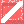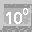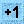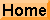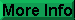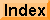## The Planck Length

The Planck length is a unit of length derived from three fundamental constants of physics: the speed of light in a vacuum, c; the gravitational constant, G; and the reduced Planck constant, ħ=h/2π. The Planck length is defined as

##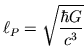Based upon the known values of c, G, and ħ, the Planck length is about 1.6 x 10-35 m.

In string theory and in quantum gravity theory, the Planck length appears to be as small as it gets. Divide the Planck length by the speed of light, c, and you get the Planck time, the shortest measurable duration of time. One can also calculate a minimum area, volume, mass, and energy at the Planck scale.